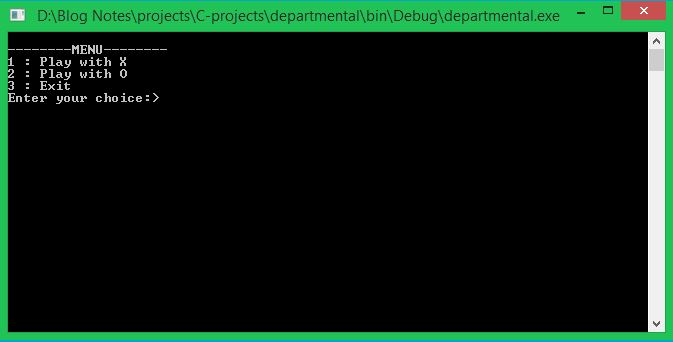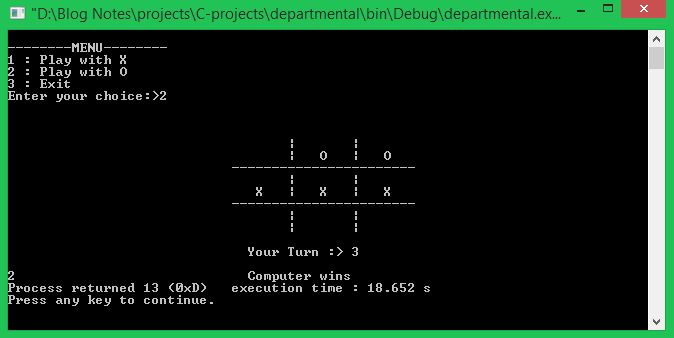# C Projects Source Code - Tic Toc Toe Game

Published October 22, 2021

In this c projects source code series we will create simple TicTacToe game with C Language. It is one of the games that most of us have played during our school days, and this game takes you back to the good old memories at your school.
Tic Tac Toe game is a c console-based application that doesn't use graphics. It is the same as the noughts and crosses (another name is 'Xs' and 'Os'). The game is built using Code:: Blocks with GCC compiler. In this game, there are 765 states of space complexities.
'Xs' and 'Os' are stored in different arrays, and the user has to choose either X or O during the gameComplete source code for Tic Tac Toe with C Language

 ```//-PROGRAMMING MADE SIMPLE #include #include #include #include int board = {2,2,2,2,2,2,2,2,2,2}; int turn = 1,flag = 0; int player,comp; void menu(); void go(int n); void start_game(); void check_draw(); void draw_board(); void player_first(); void put_X_O(char ch,int pos); COORD coord= {0,0}; // this is global variable //center of axis is set to the top left cornor of the screen void gotoxy(int x,int y) { coord.X=x; coord.Y=y; SetConsoleCursorPosition(GetStdHandle(STD_OUTPUT_HANDLE),coord); } void main() { system("cls"); menu(); getch(); } void menu() { int choice; system("cls"); printf("\n--------MENU--------"); printf("\n1 : Play with X"); printf("\n2 : Play with O"); printf("\n3 : Exit"); printf("\nEnter your choice:>"); scanf("%d",&choice); turn = 1; switch (choice) { case 1: player = 1; comp = 0; player_first(); break; case 2: player = 0; comp = 1; start_game(); break; case 3: exit(1); default: menu(); } } int make2() { if(board == 2) return 5; if(board == 2) return 2; if(board == 2) return 4; if(board == 2) return 6; if(board == 2) return 8; return 0; } int make4() { if(board == 2) return 1; if(board == 2) return 3; if(board == 2) return 7; if(board == 2) return 9; return 0; } int posswin(int p) { // p==1 then X p==0 then O int i; int check_val,pos; if(p == 1) check_val = 18; else check_val = 50; i = 1; while(i<=9)//row check { if(board[i] * board[i+1] * board[i+2] == check_val) { if(board[i] == 2) return i; if(board[i+1] == 2) return i+1; if(board[i+2] == 2) return i+2; } i+=3; } i = 1; while(i<=3)//column check { if(board[i] * board[i+3] * board[i+6] == check_val) { if(board[i] == 2) return i; if(board[i+3] == 2) return i+3; if(board[i+6] == 2) return i+6; } i++; } if(board * board * board == check_val) { if(board == 2) return 1; if(board == 2) return 5; if(board == 2) return 9; } if(board * board * board == check_val) { if(board == 2) return 3; if(board == 2) return 5; if(board == 2) return 7; } return 0; } void go(int n) { if(turn % 2) board[n] = 3; else board[n] = 5; turn++; } void player_first() { int pos; check_draw(); draw_board(); gotoxy(30,18); printf("Your Turn :> "); scanf("%d",&pos); if(board[pos] != 2) player_first(); if(pos == posswin(player)) { go(pos); draw_board(); gotoxy(30,20); //textcolor(128+RED); printf("Player Wins"); getch(); exit(0); } go(pos); draw_board(); start_game(); } void start_game() { // p==1 then X p==0 then O if(posswin(comp)) { go(posswin(comp)); flag = 1; } else if(posswin(player)) go(posswin(player)); else if(make2()) go(make2()); else go(make4()); draw_board(); if(flag) { gotoxy(30,20); //textcolor(128+RED); printf("Computer wins"); getch(); } else player_first(); } void check_draw() { if(turn > 9) { gotoxy(30,20); //textcolor(128+RED); printf("Game Draw"); getch(); exit(0); } } void draw_board() { int j; for(j=9; j<17; j++) { gotoxy(35,j); printf("| |"); } gotoxy(28,11); printf("-----------------------"); gotoxy(28,14); printf("-----------------------"); for(j=1; j<10; j++) { if(board[j] == 3) put_X_O('X',j); else if(board[j] == 5) put_X_O('O',j); } } void put_X_O(char ch,int pos) { int m; int x = 31, y = 10; m = pos; if(m > 3) { while(m > 3) { y += 3; m -= 3; } } if(pos % 3 == 0) x += 16; else { pos %= 3; pos--; while(pos) { x+=8; pos--; } } gotoxy(x,y); printf("%c",ch); } ```

 Article Contributed By :489 Views
Projects By category
Categories